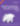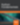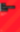# Operators in C, Part 3

Programming

Share:

#### Free JavaScript Book!Write powerful, clean and maintainable JavaScript.

RRP \$11.95

This article is a continuation of the tutorial Operators in C Part 2. It will discuss the next unary operators, the cast operator, the multiplicative and additive operators, the bitwise shift operators and the relational operators.

## Unary Arithmetic Operators

There are four unary arithmetic operators:

1. Unary minus (-)

2. Unary plus (+)

3. Bitwise Complement (~)

4. Logical Negation Operator (!)

### Unary Minus Operator

The unary minus operator precedes a numerical constant, variable or expression and negates its value. It is different from the subtraction operator (-) which requires two separate operands.

The operand of the unary minus operator must belong to the arithmetic data type domain. The arithmetic type domain consists of the Integer data types and the Floating point data types. The Integer data types consists of `char`, the `signed `and the `unsigned integer types `and the `enumerated types`. The floating point types are `float and double` types. Each of these arithmetic types belong to one type domain — the real type (stores values that are real numbers) or the complex types (stores values that are complex numbers).

### Unary Plus Operator

The unary plus operator also precedes a numerical constant, variable or expression. The result of the unary (+) operator is the value of its operand. The operand of the unary (+) operator should also belong to the arithmetic type domain.

### Bitwise Complement Operator

The bitwise complement operator (~) causes the bits to get inverted or reversed; ie, 1s become 0s and 0s become 1s. The operand must be an integer type quantity (char, signed and unsigned integer types and the enumerated types).

### Logical Negation Operator

The logical negation operator (!) negates the value of a logical expression; ie, it causes an expression that is originally true to become false and vice versa. The result of the logical negation operator has type `int`. The result of  ! is 0 if the value of the operand, which is a logical expression, is unequal to 0, and the result is 1 if the value of the operand is equal to 0.

The operand of the logical negation operator must belong to a scalar type. The scalar type domain consists of the arithmetic types (Integer and Floating types) and the pointer type.

Example: suppose i and f are integer variables where `i=7` and `f=5`, then the true logical expresion `(i>f)` becomes false when the logical negation operator is applied to it; ie, !(i>f)  evaluates to 0(false).

## The sizeof and alignof Operators

The sizeof operator yields the memory occupied by its operand in bytes. The operand may either be an expression or a data type name.

Syntax: `sizeof(operand);`

The data type can be one of the integer types , a pointer type or even a structure or union type. When sizeof is applied to an operand with type `char`, `signed char` or `unsigned char` the result is 1. If it is applied to an array, the result is the total number of bytes required by the array elements. If it is applied to a structure/union the result is the sum of the number of bytes required by each member of the structure/union. The value of the result is implementation-defined and its type (an unsigned integer type) is `size_t` defined in `stddef.h `(and other header files).

The alignof operator yields the alignment required of its operand type. An alignment is an implementation-defined integer value representing the number of bytes between successive addresses at which a given object can be allocated. The data type of an object imposes an alignment requirement on every object of that type. Stricter alignment can be requested using the` _Alignas` keyword.

A fundamental alignment is less than or equal to the greatest alignment supported by the implementation (the target machine/platform) which is equal to` alignof(max_align_t)`.

An extended alignment is greater than `alignof(max_align_t)`. It is implementation-defined whether extended alignments are supported or not.Make the leap into server-side programming with a comprehensive cover of PHP & MySQL.

Normally RRP \$11.95 Yours absolutely free

Alignments are represented as values of type `size_t`. Valid alignments include only those values returned by an `alignof` expression for fundamental types, plus an additional implementation-defined set of values, which may be empty. Every valid alignment value shall be an integral power of two.

The sizeof  and alignof operators cannot be applied to an expression that has function type or an  incomplete data type (such as a void type or to a variable length array), to the parenthesized name of such a type, or to an expression that designates a bit-field member.

## The Cast Operator

The value of an expression can be converted to a different data type if desired. To do so, the expression must be preceded by the name of the desired data type enclosed in parantheses, ie:

`(data type) expression`

This type of construction is known as a cast.

Example: Suppose that` i` is an integer variable whose value is 7, and `f` is a floating point variable whose value is 8.5. The expression` (i+f)%4` is invalid because the first operand is floating point and the `%` operator works only on integer operands. However, the expression,` ((int) (i+f))%4 `forces the first operand to be an integer, and is therefore valid. Thus, the  output of this expression is 3 as the % operator gives the remainder after integer division. Note that the explicit type specification applies only to the first operand and not to the entire expression.

The data type associated with the expression itself is not changed by a cast. Rather, it is the value of the expression that undergoes type conversion wherever the cast appears. This is particularly relevant when the expression consists of only a single variable.

## Multiplicative Operators

There are three multiplicative operators:

### Multiplication operator (*)

The * operator gives the product of two operands. The operands must be of arithmetic data type.

### Division operator (/)

The / operator produces the quotient after dividing the first operand by the second. The two operands must be of arithmetic type and the second operand must be non-zero.

### Modulus operator (%)

The % operator produces the remainder after division. The operands of the % operator must be integers and the second operand must be non-zero.

There are two additive operators in C:

The + operator performs binary addition and produces the sum of the two operands. The two operands of the + operator should both be of arithmetic type, or otherwise one operand should be a pointer  type and the other should  be of integer type.

### Subtraction operator (-)

The – operator subtracts the second operand from the first and gives the difference. For subtraction, the data types of the operands must satisfy one of the following combinations:

1. Both operands should be of arithmetic type.
2. Both operands should be a pointer to a complete object type.
3. The left operand should be a pointer to a complete object type and the right operand should be of integer type.

Operations on pointers will be discussed when we discuss pointers in a later article.

## Bitwise Shift Operators

The two bitwise operators are shift left (<<) and shift right (>>). Each operator requires two operands. The first is an integer type operand that represents the bit pattern to be shifted. The second is an unsigned integer that indicates the number of displacements (ie, whether the bits in the first operand will be shifted by 1 bit position, 2 bit positions, 3 bit positions etc). This value cannot exceed the number of bits assosciated with the word size of the first operand.

The left shift operator causes all of the bits in the first operand to be shifted to the left by the number of positions indicated by the second operand. The leftmost bits (ie the overflow bits) in the original bit pattern will be lost. The rightmost bit positions that become vacant will be filled with 0s.

Example: Suppose` a` is an unsigned integer variable whose value is `0110 1101 1011 0111`. The expression

`b = a<<6`

will shift all bits of` a` six places to the left and assign the resulting bit pattern to the unsigned integer variable` b`. Therefore the resulting value of `b` will be `0110 1101 1100 0000`. All of the bits originally assigned to `a` are shifted to the left six places. The leftmost 6 bits, ie `0110 11,` are lost and the the rightmost 6 bit positions are filled with 0s.

The right shift operator causes all of the bits  in the first operand to be shifted to the right by the number of positions indicated by the second operand. The rightmost bits (ie, the underflow bits) in the original bit pattern will be lost. If the bit pattern being shifted represents an unsigned integer, then the leftmost bit positions that become vacant will be filled with 0s.

Example: Suppose `a` is an unsigned integer variable whose value is `0110 1101 1011 0111`. The expression

`b= a>>6`

will shift all bits of  `a` six places to the right and assign the resulting bit pattern to the unsigned integer variable `b`. Therefore the resulting value of` b` will be `0000 0001 1011 0110`. The rightmost 6 bits of a,  ie` 11 0111, `are lost. The leftmost 6 bit positions are filled with 0s.

## Relational Operators

There are four relational operators in C:

1. Less than (<)

2. Greater than (>)

3. Less than or equal to (<=)

4. Greater than or equal to (>=)

Relational operators are used to form logical expressions, which represent conditions that are either true or false. The resulting expressions will be of type integer, since true is represented by the integer value 1 and false by 0.

In a logical expression that uses relational operators either both the operands are of real data type or both are of pointer type.

When two pointers are compared, the result depends on the relative locations in the address space of the objects pointed to. If two pointers point to the same object, or both point one past the last element of the same array, they compare equal. If the objects pointed to are members of the same structure/union, pointers to structure members declared later compare greater than pointers to members declared earlier in the structure, and pointers to array elements with larger subscript values compare greater than pointers to elements of the same array with lower subscript values. All pointers to members of the same union object compare equal.

Example: Suppose `i` and` j` are integer variables where `i=3` and `j=5`. Then the logical expression `i>j` will evaluate to false,  ie 0, and the expression`(i+5)>=j` will evaluate to true, ie 1.

The next article on C will continue with the rest of the operators: equality operators, bitwise AND, bitwise OR, logical AND, logical OR, conditional operators, assignment operators and the comma operator.### Surabhi Saxena

My name is Surabhi Saxena, I am a graduate in computer applications. I have been a college topper, and have been awarded during college by the education minister for excellence in academics. I love programming and Linux. I have a blog on Linux at www.myblogonunix.wordpress.com.

#### New books out now!Learn valuable skills with a practical introduction to Python programming!Give yourself more options and write higher quality CSS with CSS Optimization Basics.